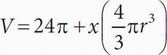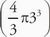# SAT Math Multiple Choice Question 66: Answer and Explanation

### Test Information

Question: 66

6. Jeff tests how the total volume occupied by a fluid contained in a graduated cylinder changes when round marbles of various sizes are added. He found that the total volume occupied by the fluid, V, in cubic centimeters, can be found using the equation below, where x equals the number of identical marbles Jeff added, one at a time, to the cylinder, and r is the radius of one of the marbles.If the volume of the graduated cylinder is 96π cubic centimeters, then, what is the maximum number of marbles with a radius of 3 centimeters that Jeff can add without the volume of the fluid exceeding that of the graduated cylinder?

• A. 1
• B. 2
• C. 3
• D. 4

B This is a good Plug In the Answers problem. Start with (B) and plug in 2 for x and 3 for r in the equation to get V = 24π + 2, which is equal to the target amount of 96π, so (B) is correct.